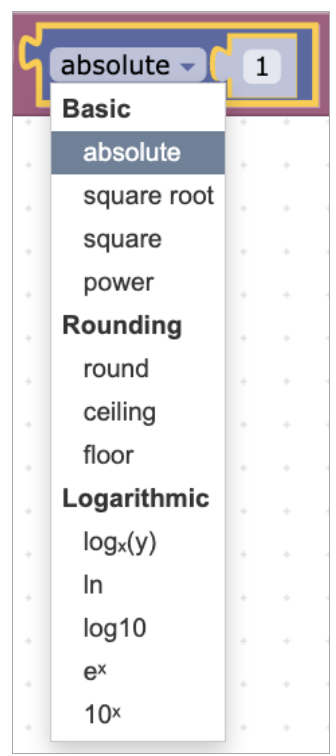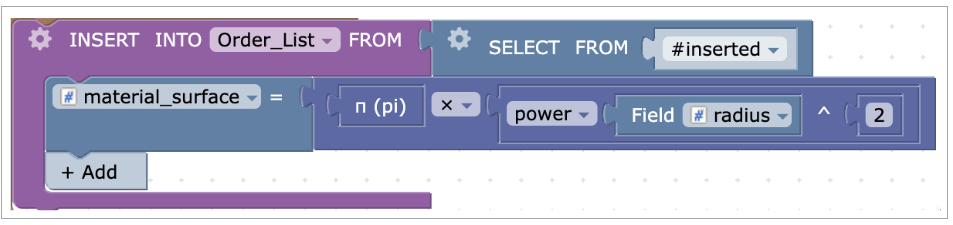Mathematical operators and static constants including basic arithmetic and random numbers.

#### Numeric field

Fixed value for any number. It can be used to assign a static value to a table field or any other place that accepts a number datatype.#### Mathematical operations

Mathematical operations such as addition, subtraction, division, multiplication, and operations with modulo (%) that return the remainder of a division operation.#### Negative number

Negative value negating the given value. It can be used to create an inversed (opposite) number that can be employed in calculations or file field assignments.#### Arithmetic operations, floor and ceiling functions, logarithms

Arithmetic, rounding, and logarithmic mathematical operations and processes.The following options are available:

• Absolute - returns the absolute value of a number. For example, absolute from (-200) is 200.
• Square root - returns the square root of a number. For example, the square root from 64 is 8.0.
• Square - returns the square of a number. For example, the square of 64 is 4096.0.
• Power – returns the value of a number raised to the power of another number. For example, the power of (4, 2) is 16.
• Round - rounds the number to 2 decimal places. For example, the round of 235.415 to 2 decimal places is 235.420. Negative argument for decimal places rounds the integer part. For example, the round of 235.415 to -2 decimal places is 200.00.
• Ceiling – returns the smallest integer value that is greater than or equal to a number. For example, the ceiling of 81.75 is 82.0.
• Floor - returns the largest integer value that is smaller than or equal to a number. For example, the floor of 81.75 is 81.
• logx(y) - returns the logarithm of a number by the specified base value. For example, log of 2 to a base 4 is 0.5.
• In - returns the natural logarithm of a number. It is logarithm to the base of the mathematical constant e.
• log10 - returns the decimal logarithm of a number. It is a logarithm to the base of 10. For example, log(10)2 is 0.3010299956639812.
• ex - returns math constant e raised to the power of a specified number. For example, e raised to the power 2 is 7.3890560989306504.
• 10xreturns 10 raised to the power of a specified number. For example, 10 raised to the power 3 is 1000.The above example presents geometrical mean calculated from all records in the Transactions table according to the following formula:#### Random number

Random number returns a random decimal fraction, that is the number between 0 (inclusive) and 1 (exclusive).The above triggered action generates a coupon code for a newly inserted client by using a random number block and sets the expiration date to one year. Then, it sends an email to the client with a notification that the coupon is available.

#### Sign of

Sign of returns the sign of a number as follows:

• If number > 0, it returns 1
• If number = 0, it returns 0
• If number < 0, it returns –1

It can be used to analyze the month-over-month change in a company's net income. If Sign of returns 1, it indicates a positive growth in net income; if it returns 0, there is no change; and if it returns -1, there is a decline in net income.#### N(pi)

N(pi) returns Pi number value.The above trigger calculates the surface area of a circle (πr²) that is required for ordering purposes.